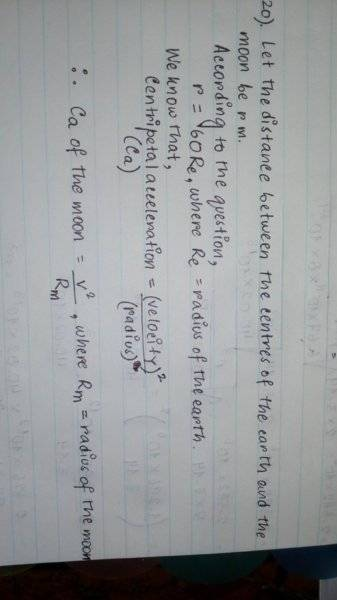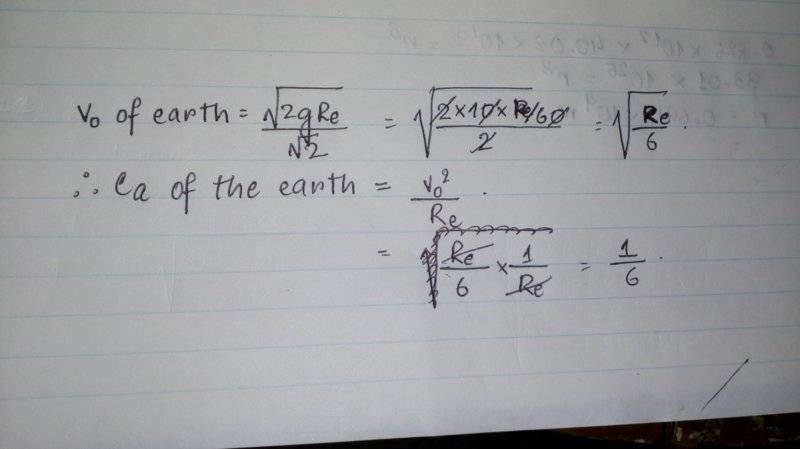# Gravitation sum related to Centripetal Acceleration

• Urmi
In summary, the conversation discussed calculating the centripetal acceleration of the moon, which is 60 times the radius of the Earth away from the Earth's center. The equation for centripetal acceleration was given, as well as the equation for orbital velocity. The gravitational acceleration at the Earth's surface was also mentioned, and it was explained how to find the acceleration of the moon using the Earth's gravitational field. The final equation was derived and it was determined that the centripetal acceleration of the moon is equal to the gravitational acceleration of the Earth's field at a distance of 60 times the Earth's radius.f

## Homework Statement

The distance between the centres of the Earth and the moon is 60 times the radius of the earth. Calculate the centripetal acceleration of the moon. Acceleration due to gravity on the Earth's surface is 10m/s.

## Homework Equations

Centripetal acceleration= v^2/R
Orbital Velocity=√2gR/√2

## The Attempt at a Solution

I found out the centripetal acceleration of Earth but I cannot find that of the moon.#### Attachments

Firstly, notice that they did not give you the mass of the earth(Me) which is necessary if you want to find the acceleration due to the Earth's G-Field.
a = GME/r^2
However, you don't need the actual mass of the Earth since a is proportional to r^(-2) (a = kr^(-2)). Hope this helped. :)
P.S. This is all assuming that the G-Field of the moon is negligible for an object on Earth's surface.
Umm, I'm sorry...can you just elaborate on the answer, like how to find the a?? I'm confused a bit...

The centripetal acceleration of the moon equals the gravitational acceleration of the Earth's gravitational field at distance ##r=R_m=60R_e##.
The gravitational acceleration of Earth's gravitational field at distance ##r=R_e## (that is at the surface of the earth) is given equal to ##10m/s^2##

The gravitational acceleration of the Earth's gravitational field at a specific distance r is given by ##a_r=\frac{GM_e}{r^2}##. Apply this equation first for ##r=R_e ## and then for ##r=R_m=60R_e##. So you ll get two equations. Divide the two equations by parts, the ##GM_e## will be simplified, and you ll get an equation with only one unknown, ##a_{R_m}## that is the gravitational acceleration due to Earth's gravitational field at distance ##r=R_m##.

•Urmi
The centripetal acceleration of the moon equals the gravitational acceleration of the Earth's gravitational field at distance ##r=R_m=60R_e##.
The gravitational acceleration of Earth's gravitational field at distance ##r=R_e## (that is at the surface of the earth) is given equal to ##10m/s^2##

The gravitational acceleration of the Earth's gravitational field at a specific distance r is given by ##a_r=\frac{GM_e}{r^2}##. Apply this equation first for ##r=R_e ## and then for ##r=R_m=60R_e##. So you ll get two equations. Divide the two equations by parts, the ##GM_e## will be simplified, and you ll get an equation with only one unknown, ##a_{R_m}## that is the gravitational acceleration due to Earth's gravitational field at distance ##r=R_m##.
Thanks a ton! It helped a lot :)

•Delta2
@Urmi, in future, please be advised that you cannot post images of handwritten equations. You need to use the PF LaTeX feature to post math directly in the thread. See here for help:

https://www.physicsforums.com/help/latexhelp/
Okayy, I'm new here so I never knew this...I'll be sure to use this next time :)

•berkeman